Critique of Shawn Bishop’s Paper “In Disdain of Garbage Physics”.

©Cyril Smith, March 2003

1.  Introduction

Shawn Bishop has published a paper1 debunking the claims of Tom Bearden regarding the Motionless Electromagnetic Generator (MEG)2 and Jean-Louis Naudin’s replication of this device3.  This paper is my response to Bishop, demonstrating that his arguments are seriously flawed.

2.  Some Basic Physics Theory

I have used the above section heading to replicate that used by Bishop.  Having previously used I0 to signify the peak value for a sinusoidal current, in his third paragraph he states:-

“For AC circuits, the relevant quantity for power is the root-mean-square (RMS) power over one complete cycle of the current and/or voltage.  If the voltage, V, is constant and the current is a sinusoidal AC, the RMS power over one cycle, Prms, is given by,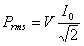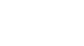(3)”

(his equation 3)

This statement is both misleading and confusing.  The use of an RMS value on a cyclic power waveform is incorrect.  In electronics the math function RMS is correctly used to express the power capability of a sinusoidal voltage or current waveform (assuming that the load is purely resistive).  As the RMS name implies, the waveform is first squared so as to become a power waveform, then the mean value of the power waveform is taken, finally the square root of this mean value is used so as to arrive at the effective DC-power value for the original current or voltage.  Note the mean value of the power waveform.  It would be quite wrong to use the math RMS function on the squared (power) waveform.

The fallacy of Bishop’s equation (3) is easily seen by examination of his power waveform.  A constant voltage V multiplied by a sinusoidal current I0sin(wt) yields a power waveform VI0sin(wt) which remains a pure sinusoid.  The positive half cycle represents forward power flow, the area under the curve being the total forward energy transported during that half cycle.  The negative half cycle represents reverse power flow, and again the area represents the total energy transported.  Symmetry of the sinusoid shows that over a full cycle the net energy transported is zero.  The correct math function to apply to this power waveform is mean or average.  Bishop’s equation (3) is wrong, it should be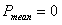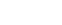Bishop(3).

That would give Bishop some difficulty in arriving at his equation (5) which correctly gives the mean power (but labels it Prms) for a voltage sinusoid multiplied by a current sinusoid, but his whole method for deriving this is wrong.  Taking V0 as the peak value for the voltage, the voltage × current (power) waveform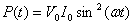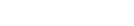can be rewritten as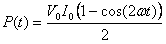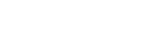which has a mean value of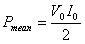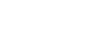Bishop(5)

3.  Looking Closely at the Data

Bishop’s close(!!) look at Naudin’s data states

• The input voltage is constant and is about 28 V.
• The input current is AC (not perfectly sinusoidal but close enough).
• The amplitude of the AC current, I0, is about 125 ×10-3 (take the difference in the peak-to-peak values and divide by two).

He later uses his flawed equation (3) to calculate input power as 2.47±0.33 watts.  Had he used the correct value of zero, he might have been drawn to the fact that he had overlooked the position of the zero current line on the scope trace.  The input current is not simple AC, it is AC stood on a DC level.  Naudin’s scope correctly takes the product of the DC voltage and this DC+AC current to display the power waveform, and Naudin correctly uses the mean value of this power waveform as input power.

Bishop makes another mistake with regard to his calculation of output power.  In spite of evidence to the contrary, and Naudin’s clear statement that the resistor is conditioned, he takes Naudin’s load resistor at its face value of 100K.  Naudin gives full details of the conditioning process, and although one might consider this a strange way to obtain a non-linear resistor, one thing is for sure, after that treatment it is no longer 100K!  Naudin’s own voltage/current measurements show this, but Bishop insists on using the 100K value then claims Naudin’s current measurement to be wrong by a factor of nine.  I consider that logic to be inconsistent.

4.  Perpetual Motion

Bishop is obviously of the opinion, held by many in the scientific fraternity, that perpetual motion machines are impossible.  Stone-age man would hold this opinion with regard to windmills, but today we fully accept that, in a perpetual wind, a windmill will provide useful perpetual motion.  This is because we can see and understand the source of the driving energy.  Well, consider this.

It is known that the field from a permanent magnet comes from certain electron orbital motion or spins, and we might note that these motions are perpetual.  It is also known that these electrons motions, in a magnetic field, give rise to precession at the Larmor frequency (known as electron-spin resonance or ferro-magnetic resonance).  We do not normally have access to the Larmor frequency because (a) it is in the microwave band, (b) there is a spread of frequencies and (c) there is no general phase-coherence.  Nevertheless we do have something like an array of precessing gyroscopes, possessing mechanical energy stored in their precession.  When you calculate the quantity of energy stored in this precession you find it to be of the same order as the energy of the magnetic field inside the magnet.  Thus Bearden’s NdFe magnet, quoted as 40mm × 25.4mm × 38.1mmm, has stored within it something like 30 joules of mechanical energy.  If, in each cycle of MEG operation, only 0.01% of this energy were extracted, then at the MEG frequency of 25KHz we would get 75 watts out.

Removing precession energy involves altering the precession angle which is determined by quantum rules.  Is it possible that the zillions of photons, sub-photons and virtual photons which form the energetic vacuum, the Dirac sea, the very raison d’etre for the quantum rules, could restore the precession angle, could make good the extracted energy?

5.  Conclusion

Bishop’s paper is flawed on several counts

• His equation (3) is incorrect.
• He has not included the DC component of the input current.
• He has not used the actual load resistance value.

For these reasons his calculations are not valid and should be ignored.

1 Shawn Bishop, In Disdain of Garbage Physics, (Dated: March 5, 2003)

2 Patrik et al, US Patent 6,362,718 B1, Motionless Electromagnetic Generator.

3 J L Naudin, JLN Labs: The MEG Project, http://jnaudin.free.fr/meg/meg.htm